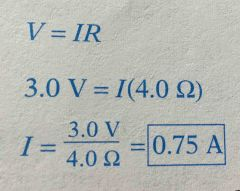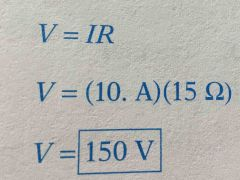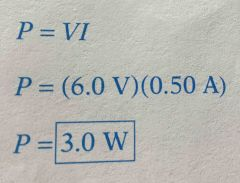• Shuffle
Toggle On
Toggle Off
• Alphabetize
Toggle On
Toggle Off
• Front First
Toggle On
Toggle Off
• Both Sides
Toggle On
Toggle Off
Toggle On
Toggle Off
Front

## Card Range To Study

throughPlay buttonPlay buttonProgress

1/44

Click to flip

Use LEFT and RIGHT arrow keys to navigate between flashcards;

Use UP and DOWN arrow keys to flip the card;

H to show hint;

 What is the SI unit of resistance? Ohm What is the SI unit of current flow? Ampere What is SI unit is used to measure the "force" with which electric current flows? Volt What type of material allows electric current to flow through it with no resistance at all? Superconductor A material through which current does not flow easily is a ____. Insulator A circuit containing a gap that electrons cannot cross is a ____ circuit? Open The ability of magnetism to convert mechanial motion into electrical current is electromagnetic __?__. induction A lamp that produces light by heating a filament until it glows is a __?__. incandescent What type of electric discharge lamp produces light by passing an electric current through a long tube filled with low-pressure mercury vapor? fluorescent lamp A circuit with loads arranged in separate branches of the current is divided among them is a ___?___ circuit. parallel automatic switch that opens a circuit when the current exceeds a predetermined amount circuit breaker allows communication over long distances using electromagnets telegraph converts pulses of electricity into sound waves loudspeaker increases or decreases the voltage of alternating current using electromagnetic induction transformer uses electromagnetic induction to produce electric current generator houses a small metal strip that breaks halt excessive current fuse semiconductor that emits light when a direct electric curren is applied light-emitting diode group of electrochemical cells connected as a single source of electricity battery electrical switch operated by an electromagnet relay simple device that converts electricity to back-and-forth motion solenoid The parts of a simple electric circuit are a source of __?__, a conductor, and a __?__. currentload The "force" with which electricity flows is called __?__. voltage The rate of charge flow is called __?__. current An electrical device designed to add resistance to a circuit is a __?__. resistor Any device used to open and close a circuit is a __?__. switch The four factors that affect resistance are the type of material, the __?__ of the object, the length of the object, and the __?__. diametertemperature Arranging electrochemical cells in parallel causes the voltage to __?__. remain the same Which of the following does not affect the strenth of an electromagnetically induced current? length of a magnet What unit do electric companies use to charge for electricity? kilowatt-hour Which of the following is not a part of a simple AC motor? commutator What is the total voltage in a series circuit? sum of individual voltages The amount that an object hinders electron flow is __?__. resistance Electric current that flows in both directions is __?__ current. alternating The energy expended to overcome "electrical friction" is converted to __?__ heat. Joule A generator that uses a fluid as a moving conductor is a __?__ generator. magnetohydrodynamic The situation in which electricity takes a chortcut through the circuit is a __?__. short circuit According to Ohm's Law, current and voltage are __?__ proportional. directly If the current in a circut increases, the power __?__. decreases A wire moving in a magnetic field generates no current if it travels __?__ to the lines of flux. parallel The relationship between voltage, current, and resistance is __?__. Ohm's How much current does a flashlight use if it's battery supplies 3.0 V and its bulb has a resistance of 4.0 ohm?A heating element has a resistance of 15 ohm's and can handle 10. A of current. What is maximum voltage it can be connected to before it will burn out?Essay:Why is a simple AC motor with a permanent field magnet not run if the motor is connected to a DC source? How does the design of a DC motor overcome this problem? The current in a motor's armature has to change direction every half turn to swap the armature's magnetic poles; otherwise, the armature will stop with its poles aligned with the poles of the field magnet. An AC motor depends on the changing direction of alternating current to change the direction of the current in the armature; the current does not change direction if the motor is hooked up to DC. A DC motor uses a commutator to switch the direction of current in the armature every half turn. How much power does a cordless phone use if it draws 0.50 A of current at 6.0 V?